# IB Manual – Capital Asset Pricing Model (CAPM)

A mathematical estimate of the cost of equity

## Capital Asset Pricing Model (CAPM)

The Capital Asset Pricing Model is a mathematically simple estimate of the cost of equity. CAPM states that investors require additional returns (risk premium) in excess of a risk-free rate proportional to market risk. It is the required return demanded by investors in a risky asset.

Cost of equity, Ke, is given by the following formula:

###### Ke = Risk free rate + (Beta * Equity Market Premium)

Where:

• Equity Market Premium (EMP) is the excess of the expected return on a market portfolio as compared to the risk-free rate.
• Beta (β) measures the level of risk of an asset relative to the risk of the overall market. If a stock is less risky than the market average, its β will be less than 1. If a stock is riskier than the market average, β will be greater than 1.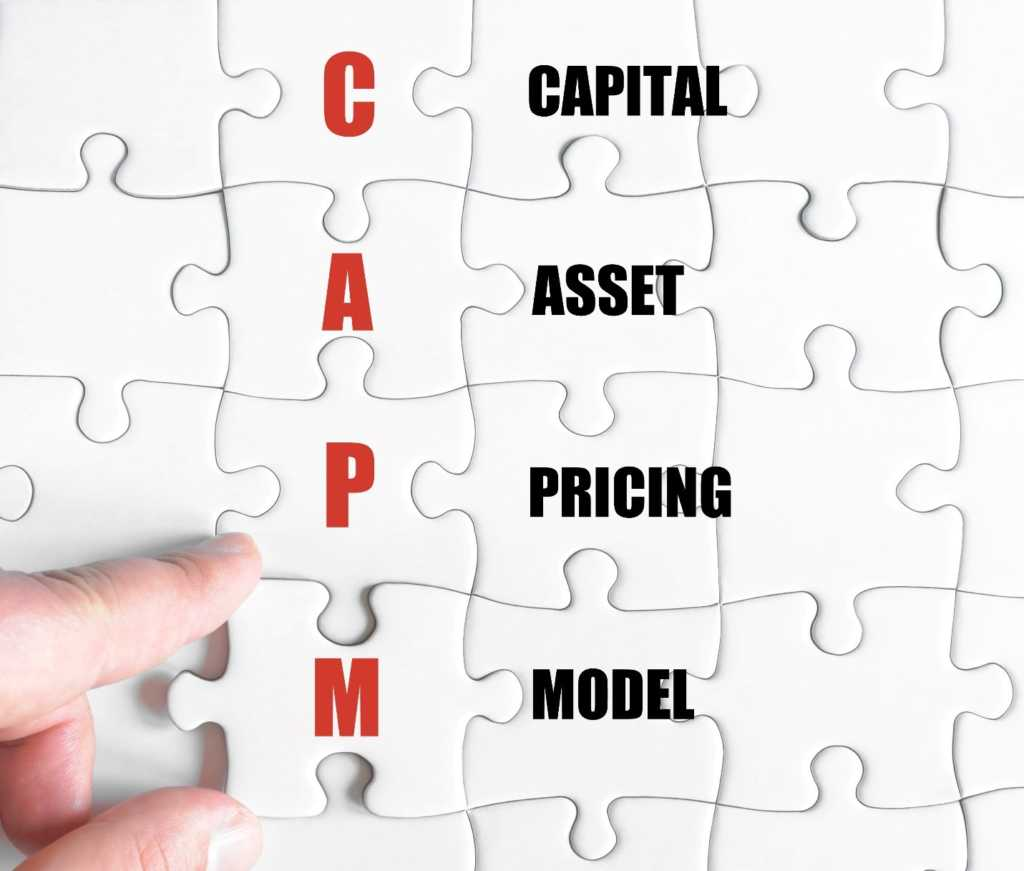### Assumptions of the CAPM

Diversification is an excellent way to shield against risk. However, diversification may include costs such as transaction costs and investment monitoring. This leads to an issue of cost-benefit analysis. Instead, the CAPM model assumes that:

• There are no transactions costs.
• Investments are infinitely divisible.
• Investors can access all information and are equally well informed.

The CAPM ignores any associated costs of diversification, and so investors will diversify until their portfolio contains all assets in the market. Therefore, all investors will diversify until they each hold the market portfolio. Every investor holds the same portfolio.

### Meaning of risk under the CAPM

The risk of an individual asset is the risk that the asset brings to the market portfolio. If the risk of the asset is firm-specific, the impact will be diversified away. However, if the asset moves with the market portfolio, it will add to the risk of the market portfolio. Covariance of an asset is the strength of related movement between an asset and the market. The risk of the market portfolio is measured as the variance in the returns on the market portfolio σ2m. The variance of the asset that is going to be added to the portfolio is σ2i.

Pre-asset inclusion portfolio variance = σ2m

Post asset inclusion portfolio variance  = wi2σ2i + (1-wi)2 σ2m + 2wi(1-wi) σim

Where:

wi = Market value weight of the new asset introduced to the portfolio

σ2m = Variance of the individual asset introduced to the portfolio

σim  = The covariance of the asset’s return with the market portfolio’s return

Covariance, σim, can be standardized by dividing it by the variance of the market portfolio.  This equation gives us the asset’s beta – a relative measure of the risk of an asset in relation to the market.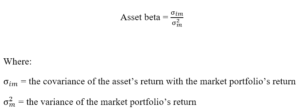The Covariance of the market portfolio with itself is its variance.

### Understanding the CAPM equation

The standard CAPM equation is:

###### E(Ri) = Rf + βi[E(Rm) – Rf]

Where:

• E(Ri) – Expected (or required) return on an asset
• R– Risk-free rate
• E(Rm) – Expected return on the market portfolio
• β– Beta of the asset

Based on this equation, we see that assets with a higher beta (more inherent risk) demand a higher expected return.

### Risk-free rates

The risk-free rate represents a theoretical rate of return with no variability (an asset beta of 0). The risk-free rate is commonly based on government bonds. While most government bonds are free from default risk, they are not totally risk-free.  Government bonds are exposed to reinvestment risk as interest rates move after the interest rates on the bonds are fixed. For this reason, there is no genuinely risk-free asset. Nevertheless, analysts, as a matter of convenience, commonly use the 10-year government bond yield as the risk-free rate in CAPM analysis.

### Beta

Common approaches to measuring beta include:

• Use historical information to perform linear regression (historical market betas)
• Estimate the beta from company fundamentals (fundamental betas)
• Estimate the beta from accounting data (accounting betas)

### Historical market betas

The regression of historic returns of an asset against the returns of the market is the standard method of producing beta estimates for firms that have been traded for a sufficiently long time. The estimated relationship between an asset and the market is the foundation for beta. Common market indexes include FTSE 100 and S&P 500.

Regression estimate for beta:

###### Ri = a+ bRm

Where:

• R– Individual stock returns
• R– Market returns
• a – Regression intercept
• b – Slope of the regression – beta

An example of a beta regression is as follows: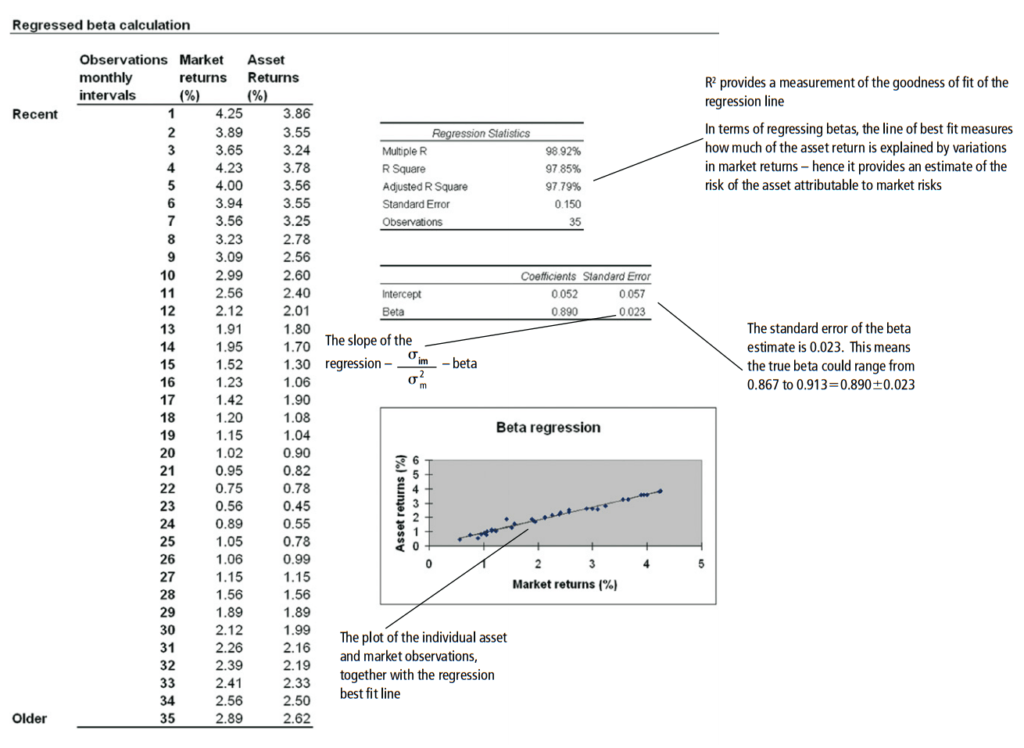Note that rearranging the CAPM equation as Ri = Rf (1-b) + βRm can be used to calculate an asset’s implied rate of return.

### CAPM – Using service betas

Running regressions for every beta is time-consuming. Fortunately, service providers such as Bloomberg give beta estimates. Before running these regressions, the following must be considered:

• Length of the estimation period (More observations may lead to bias in estimation)
• Frequency of the historic observations (More observations lead to a non-trading bias where a stock does not trade, leaving price static, but the market still moves).
• Market index used for regression (the NYSE and the S&P 500 yield different yearly returns – these differences are more apparent with more observations).

Betas are believed to revert to 1 over time, which implies that the risk of an individual project or firm equals market risk over the long run.

### Fundamental beta calculations

Beta is difficult to estimate for private companies, as their historical data is not readily available.

#### Bottom-up betas

For a bottom-up beta, betas are believed to be dependent on two factors:

• The industry the business is in
• The degree of financial leverage

These are indicators of an asset’s risk relative to the market. Bottom-up beta calculations use these factors to estimate betas from comparable information.

To conduct bottom-up beta calculations, we must:

• Identify comparable publicly traded firms and regress their historic information to obtain beta estimates
• Identifying comparable companies is difficult because many characteristics of comparability are subjectively measured. Characteristics to look for in a comparable company may include:
• Sector
• Size
• Geographical location
• Growth method (acquisition vs. organic) and growth rates
• Gearing
• Also, note that different companies are differently leveraged (using different amounts of debt). When using betas of comparable firms, we must unleverage (remove debt) the comparable company and then leverage (add debt of the firm under analysis) to reach a usable beta:
• Use the following formula to lever: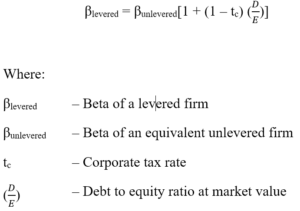• And then use the following formula to unlever: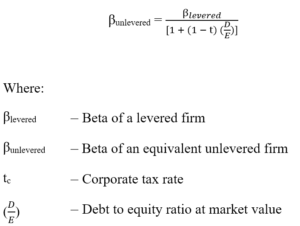To come up with a comparable beta estimate:

• Once an appropriate unlevered comparable beta’s been isolated, it will then be leveraged for the gearing of the target beta company using the equation: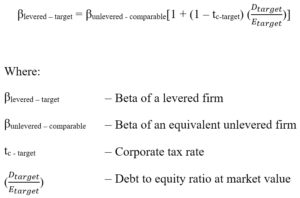Aside from not needing to depend on historical data, bottom-up betas are also useful because:

• Comparable betas are not sourced through tedious regression calculations
• They are dynamic in the sense that any business-related changes are easily modeled

### Accounting beta calculations

An accounting beta is estimated by comparing market performance against company earnings. However, calculating betas based on information that can be easily manipulated cannot yield a reliable beta.

#### CAPM – Equity risk premium [E(Rm)- Rf]

Beta measures how much the individual asset’s returns move in relation to the market portfolio and factors this into the required equity return. The CAPM needs to know the performance of a market portfolio in relation to the benchmark risk-free rate. This is given by [E(Rm) – Rf]. The CAPM takes this and quantifies the relative impact of the individual asset and, hence, derives an estimate of the required risk premium.

The premium is estimated by analyzing the historic return on stocks over the historic return of a risk-free security. The difference between the two returns yields the historic risk premium. However, there can be wide deviations, as there are differences in time length observed, the risk-free rate used, and the averaging technique used (arithmetic or geometric).

### A summary of risk/return models

Equity cost of capital can be calculated by: